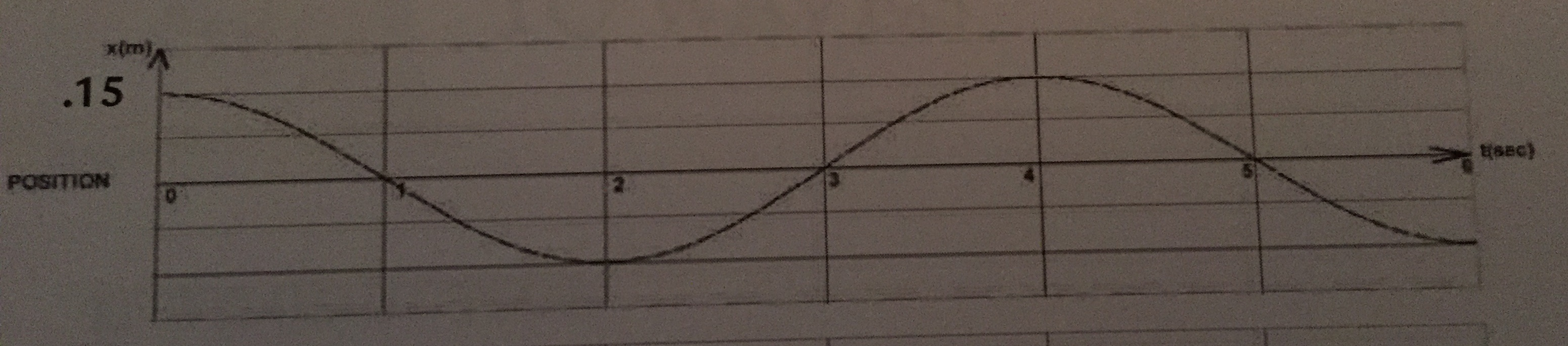# Problem: Suppose you have a block resting on a horizontal smooth surface. The block (with mass m) is attached to a horizontal spring (spring constant k), which is fixed at one end. The spring can be compressed and stretched. The mass is pulled to one side and released. The maximum displacement is 15 cm.a) If k = 150 N/m, calculate the mass of the block.b) Draw the graphs for velocity and acceleration of the motion. On the graphs, label the maximum values of velocity and acceleration, respectively.c) Draw the graph for kinetic energy and calculate and label on the graph the maximum value of the KE.d) Draw the graph for the elastic potential energy and calculate and label on the graph the maximum value of the elastic PE.e) Calculate the numerical value and draw the graph for the total energy.

###### FREE Expert Solution

In this question, we'll apply concepts from simple harmonic motion graphs and energy in simple harmonic motion.

a)

Angular frequency:

$\overline{){\mathbf{\omega }}{\mathbf{=}}\sqrt{\frac{\mathbf{k}}{\mathbf{m}}}}$

or

$\overline{){\mathbf{\omega }}{\mathbf{=}}{\mathbf{2}}{\mathbf{\pi }}{\mathbf{f}}}$

Frequency:

$\overline{){\mathbf{f}}{\mathbf{=}}\frac{\mathbf{1}}{\mathbf{T}}}$

87% (479 ratings)###### Problem DetailsSuppose you have a block resting on a horizontal smooth surface. The block (with mass m) is attached to a horizontal spring (spring constant k), which is fixed at one end. The spring can be compressed and stretched. The mass is pulled to one side and released. The maximum displacement is 15 cm.

a) If k = 150 N/m, calculate the mass of the block.

b) Draw the graphs for velocity and acceleration of the motion. On the graphs, label the maximum values of velocity and acceleration, respectively.

c) Draw the graph for kinetic energy and calculate and label on the graph the maximum value of the KE.

d) Draw the graph for the elastic potential energy and calculate and label on the graph the maximum value of the elastic PE.

e) Calculate the numerical value and draw the graph for the total energy.